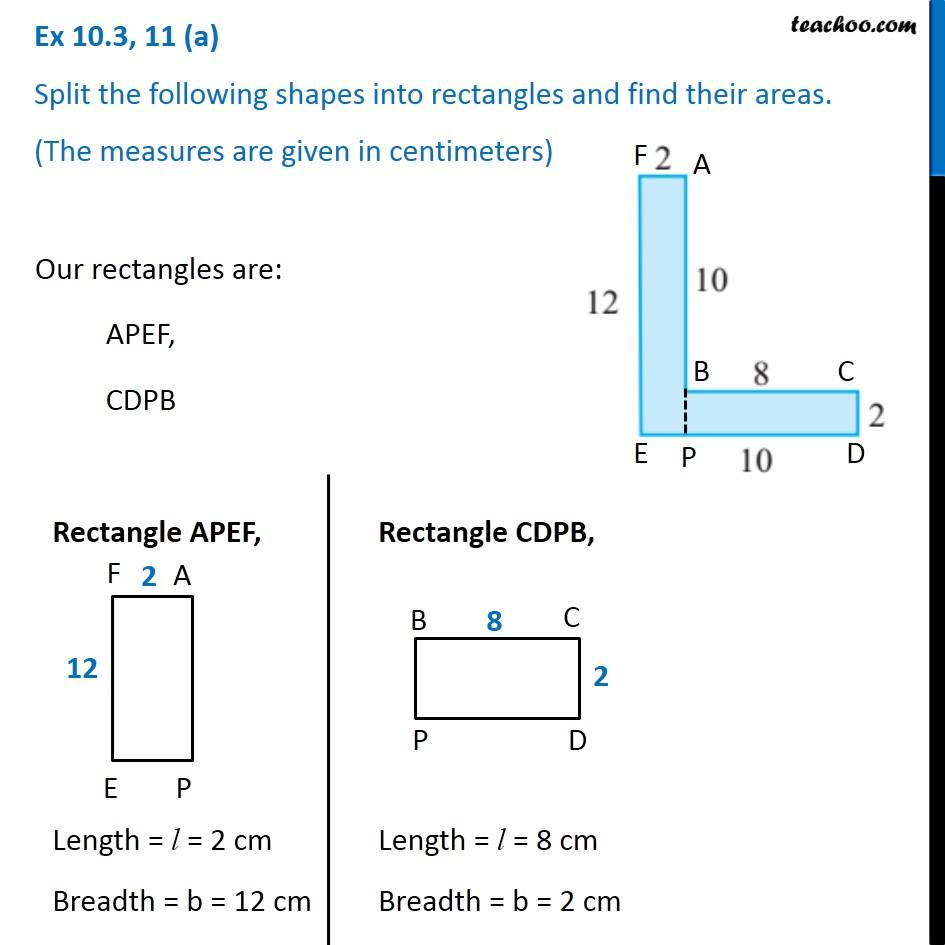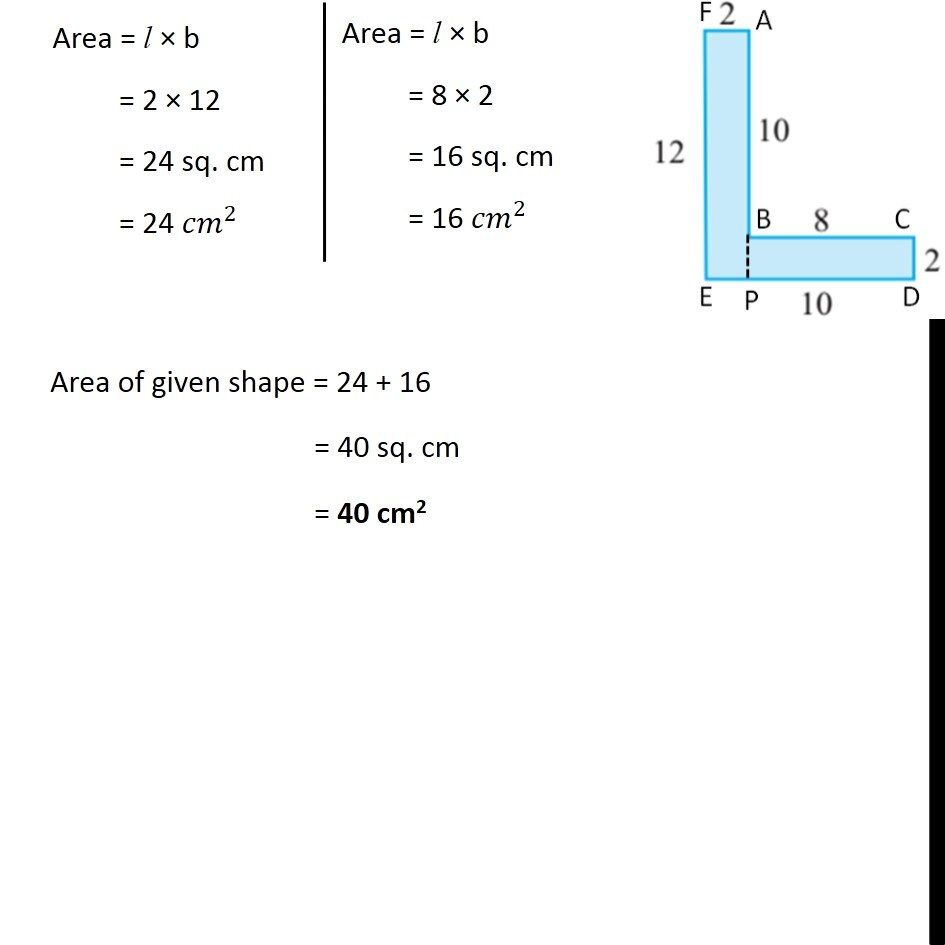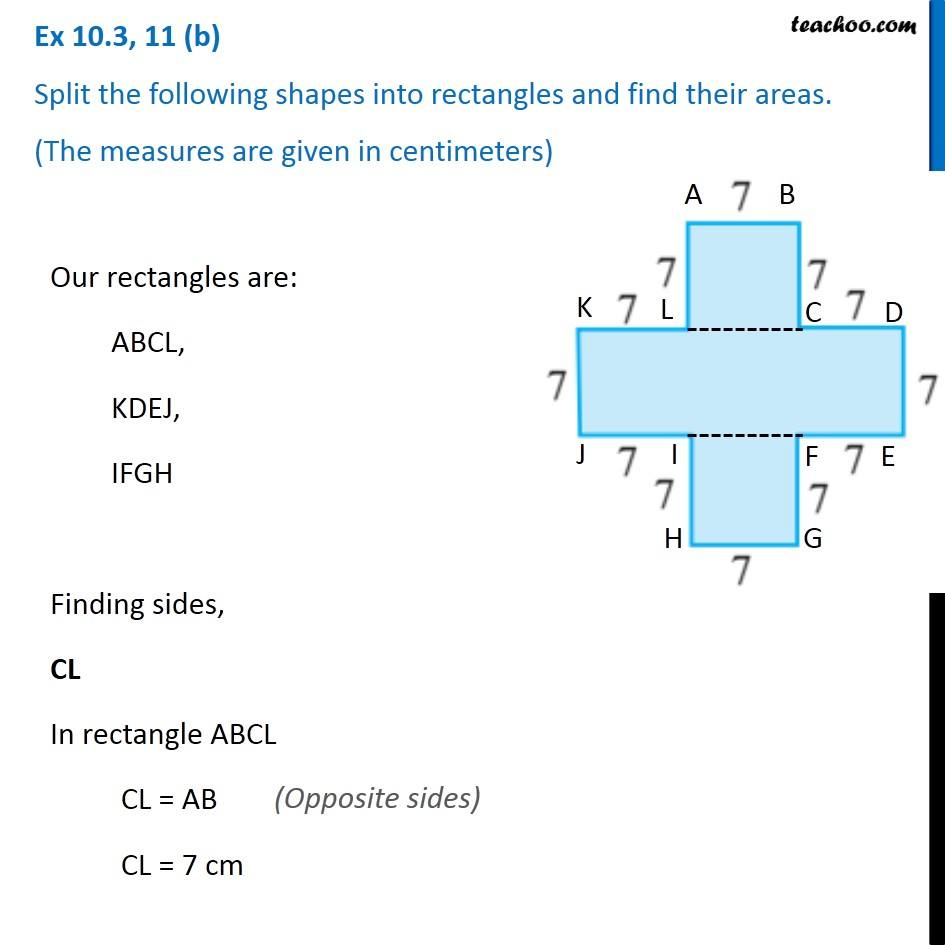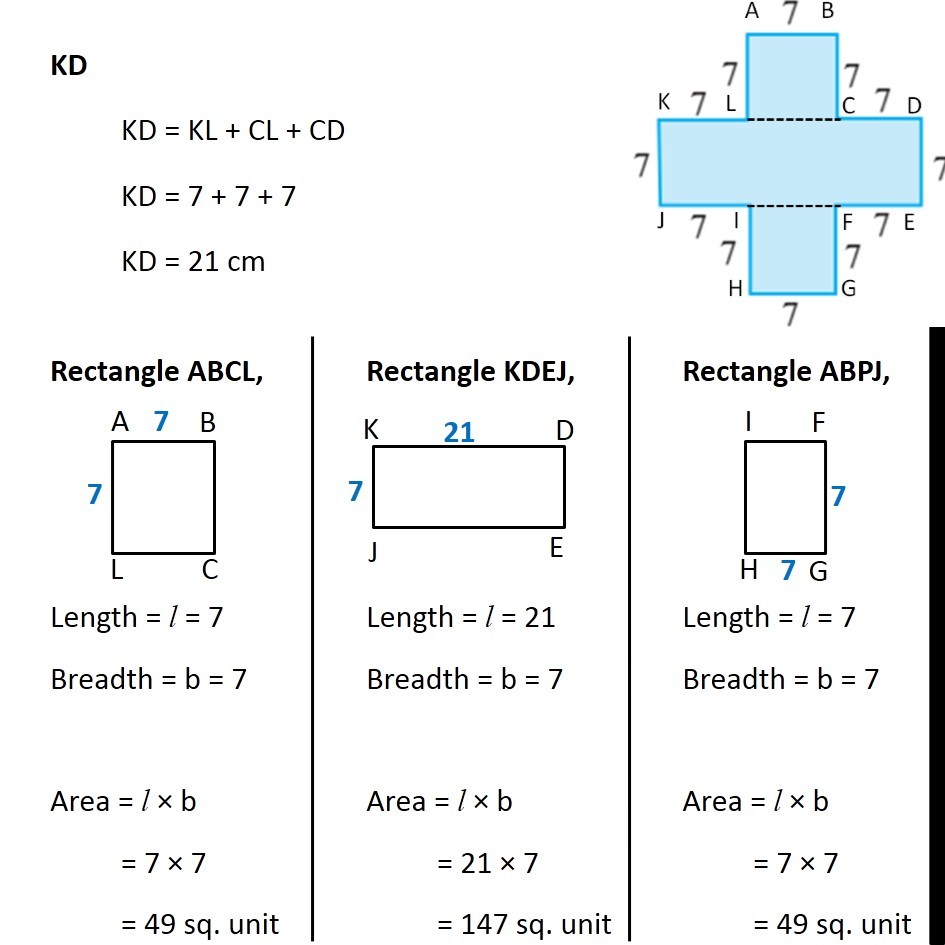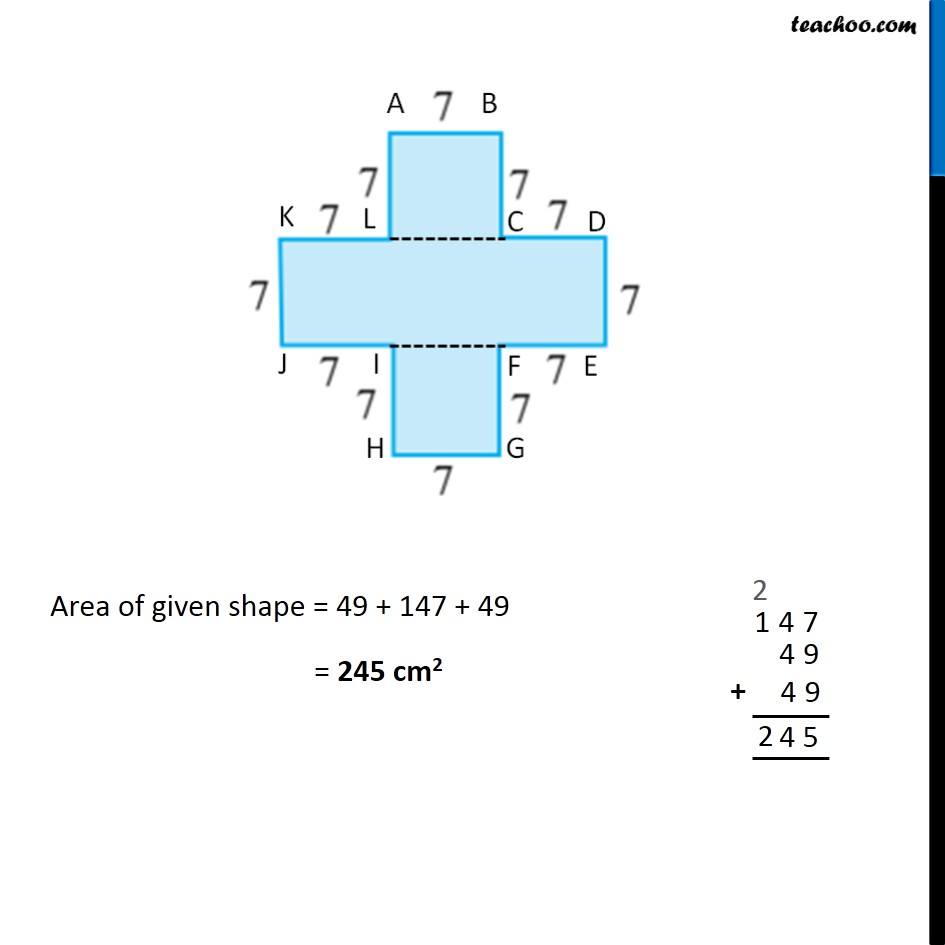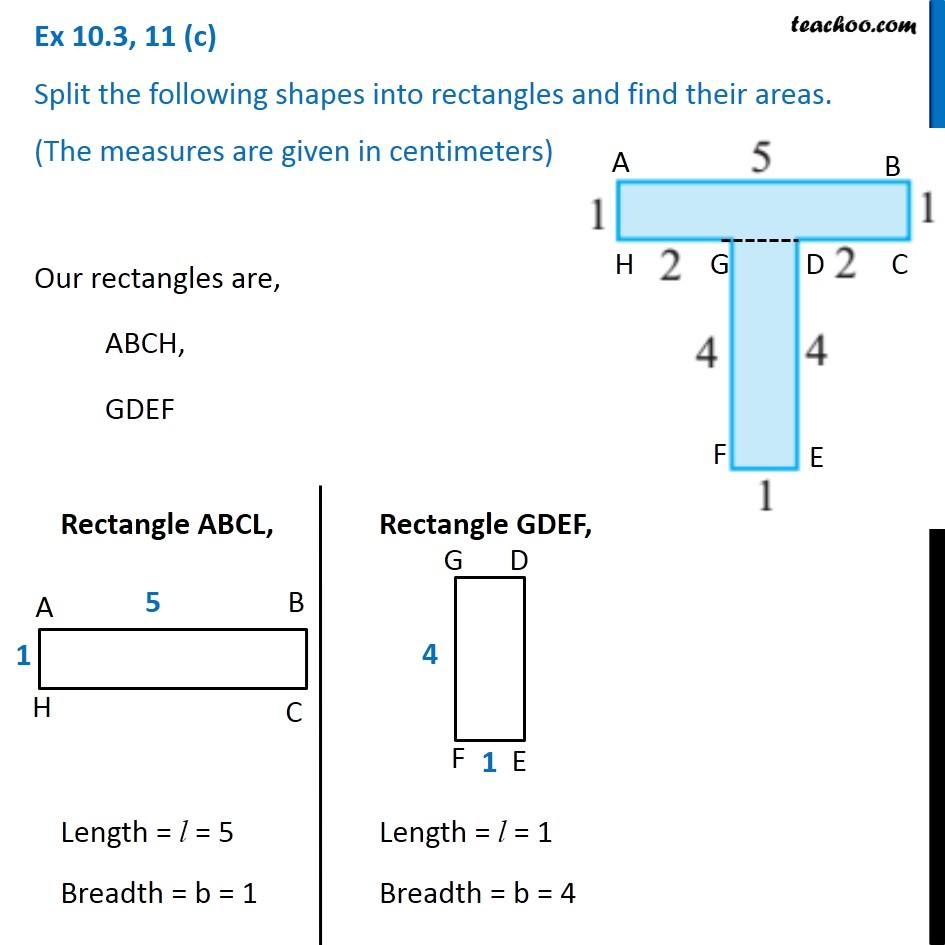Subscribe to our Youtube Channel - https://you.tube/teachoo

1. Chapter 10 Class 6 Mensuration
2. Concept wise
3. Area by splitting into rectangles

Transcript

Ex 10.3, 11 (a) Split the following shapes into rectangles and find their areas. (The measures are given in centimeters) Our rectangles are: APEF, CDPB Rectangle APEF, Length = l = 2 cm Breadth = b = 12 cm Rectangle CDPB, Length = l = 8 cm Breadth = b = 2 cm Area = l × b = 2 × 12 = 24 sq. cm = 24 〖𝑐𝑚〗^2 Area = l × b = 8 × 2 = 16 sq. cm = 16 〖𝑐𝑚〗^2 Area of given shape = 24 + 16 = 40 sq. cm = 40 cm2 Ex 10.3, 11 (b) Split the following shapes into rectangles and find their areas. (The measures are given in centimeters) Our rectangles are: ABCL, KDEJ, IFGH Finding sides, CL In rectangle ABCL CL = AB CL = 7 cm (Opposite sides) KD KD = KL + CL + CD KD = 7 + 7 + 7 KD = 21 cm Rectangle ABCL, Length = l = 7 Breadth = b = 7 Area = l × b = 7 × 7 = 49 sq. unit Rectangle KDEJ, Length = l = 21 Breadth = b = 7 Area = l × b = 21 × 7 = 147 sq. unit Rectangle ABPJ, Length = l = 7 Breadth = b = 7 Area = l × b = 7 × 7 = 49 sq. unit Area of given shape = 49 + 147 + 49 = 245 cm2 Ex 10.3, 11 (c) Split the following shapes into rectangles and find their areas. (The measures are given in centimeters) Our rectangles are, ABCH, GDEF Rectangle ABCL, Length = l = 5 Breadth = b = 1 Rectangle GDEF, Length = l = 1 Breadth = b = 4 Area = l × b = 5 × 1 = 5 〖𝑐𝑚〗^2 Area = l × b = 1 × 4 = 5 〖𝑐𝑚〗^2 Area of given shape = 5 + 4 = 9 cm2

Area by splitting into rectangles